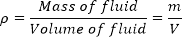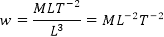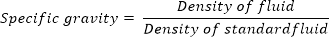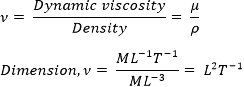Properties of Fluids in Fluid Mechanics | Mecholic -->

# Properties of Fluids in Fluid Mechanics## Mass density (ρ)

Mass density defined as the ratio of the mass of fluid to the volume of fluid. The density of liquids taken as constant while the density of gases changes with pressure and temperature.
Mass density,The SI unit of density is kg/m3
Dimensionally, ρ = ML-3

## Weight density/ specific density (w)

It is defined as the weight per unit volume. Mathematically,
Specific density,Here ρ = mass density and g = acceleration due to gravity.
The unit of specific density is N/m3
Dimension,## Specific volume

The volume of fluid occupied by unit mass. It is reciprocal of density.

Dimension: M-1L3

## Specific gravity (s)

Also known as the relative density. It is defined as the ratio of the density of the fluid to the density of the standard fluid. In case of liquid the standard fluid taken as water and for gases it is airIt is a dimensionless quantity.

## Viscosity (dynamic viscosity, µ)

It is defined as the property of a fluid that offers resistance to the fluid flow.

From newton’s law of viscosity,Dimension, µ = ML-1 T-1

## Kinematic viscosity (ν)

It is defined as the ratio of dynamic viscosity to the density of the fluid.